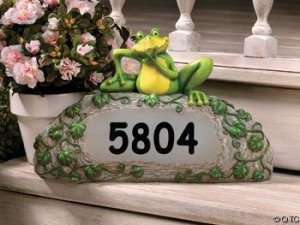Symbols / June 6, 2020

Calculate your feng shui house number to find out it's numerology significance.

According to feng shui, each number has an essence and a spiritual energy or vibration that influences the lives of those who live and work in the house.If the total number is more than 9 then add those numbers together until you reach a number nine or less...

For example, if your address is 783 Town Way, you would add 7+8+3=18; then add the numbers of the total together - 1+8=9. So the numerology would be a Nine vibration.

If you live in a block of flats and the flats has a numbered street address and your individual flat has a number, you are more affected by your individual flat number.

If your address has a letter in it, for example 328C Ivy Lane, convert the letter to a number (A=1, B=2, C=3 and so on)... So 328C Ivy Lane would convert to 3+2+8+3(c)=16; 1+6=7.

If your house doesn't have a number and only a name, you can still calculate feng shui house numbers... work out the individual letter-number equivalents and then reduce to a single number. For example, OWL HOUSE would be 6+5+3+8+6+3+1+5=37 and then 3+7=10... OWL HOUSE = 10

Now that you know the single digit vibration of your feng shui house number...